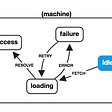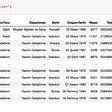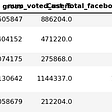# Introduction to Pandas DataFrames Part 1

Pandas is a powerful open-source library that is used in the analysis and manipulation of data. A Pandas DataFrame is a tabular representation of data.

A Pandas DataFrame is similar to an excel spreadsheet.

Now that we have a basic understanding of what Pandas is we will look at how you can start using Pandas DataFrame methods.

## Getting started

The first thing you need to do is to install Pandas. After you have had Pandas installed, we will look at Data Frames.

To begin with go to the Jupyter Lab and then we will use this data for this article.

The first step is to import `pandas as pd`. Then load the CSV file using `pd.read_csv()`.

## Checking subset of data

To view the data, write the code `df.head()` . This shows the first five rows of the data by default. If you want to see more than the first five rows, you can specify the number in the brackets. To check the last five rows of data, write the code `df.tail()` . You can also define the numbers of rows of data you want to see. This is illustrated below.

You can also view random data by writing the code, `df.sample()` .

## Obtaining data information

To check a concise summary of your data, use the `info()` method. This includes info on index and column types, non-null values, and memory usage. See this image below.

## Checking the statistical summary of the data

To check the statistical information of the data such as mean, max, count, min, use the method `describe()` .

## Selecting data in a DataFrame

The `loc[]` method is used to locate rows and columns by the labels. For instance, in this case, we can define the labels as 5 and 8. See in the screenshot below.

The method`iloc[]` is used to show the data based on the integer location that you specify. For instance in this case we can specify the location as 0.

It displays the row in the position of the integer 0. The data is displayed as series. This is because we have put one bracket.

To display it as a data frame insert two brackets.

## Checking and counting unique values

The unique method is used to return unique values from a data frame. The method is written as `.unique()`. For instance, we want to access the unique values of countries, so we will write the code as:

There is another method written as `.nunique()` which returns the number of unique values from the data series of the specified axis. Axis 0 stands for the index or rows while index 1 stands for columns. In this case, we will enter the code `df[‘country’].nunique()`that returns the number of unique countries that are there.

## Checking and filling null values

The `.isnull` function is used to return true or false (boolean)values and true for the null values. If you want to check for any values missing in a series include the `.any()` function.

## Grouping operations

When you want to group data and perform operations on the data, use the `.groupby()` function. For instance, let's say you want to group the data by sector, then perform an aggregate operation of id count. The code you will write will be as follows:

After running the code, the result will be as shown in the screenshot below.

## Sorting values

Data can also be arranged in various categories, for example, ascending and descending. In this case, let's sort the data in descending order. The code written looks like this shown below:

## Converting series to a DataFrame

To convert data from series to DataFrame, you first need to check the data type. To check the data type, write the code, `type(sectors)` . Let's check the data type of the data after running the sort values function then convert it to DataFrame.

Let's now convert the series to a data frame. You will need to reassign the data and pass it to `.reset_index()` method. The code will look like this;

In this case, lets work with five rows of data, so pass the function `head()` . The data frame will look like the image below.

## Counting values

To return data with unique rows in the DataFrame, use the `.value_counts()` method. It returns a series. The code is written as follows:

The result will look like this screenshot below;

## Saving a DataFrame

To save a DataFrame in CSV file input the code as follows :

You might also want to save the data in excel format. In that case, use the `to_excel()` method. The code will appear like the code below.

## Final thoughts

In this article, we have understood what a Panda DataFrame is and covered several methods of how we can use pandas in data analysis such as loading data, checking a subset of data, checking and counting unique values, just to mention a few. In the second part of this series, we will cover more functions such as dropping data, conditional selecting, merging files, creating new columns, applying functions, and creating pivot tables.

--

--

--

## More from Ann Wambui

Tech lover|Writer|Photographer

Love podcasts or audiobooks? Learn on the go with our new app.

## What is Virtualization?## Getting started programmatically in Swift## CSS Animations with Finite State Machines## The Pathfinder mission & multithreading issues## NFT- Weekly Digest | Week 08## Lazy yet the ‘Mysterious’ and ‘Funny’ sorting algorithm-Sleep Sort## Collapsible Scalable Silicone Folding Colander — Different Colors## Ann Wambui

Tech lover|Writer|Photographer

## How to use some pandas’ arguments, functions, and methods?## Importing data and cleaning with Pyhton## Beginner’s Pandas## Troubles I had when switching from R to Python and which way worked for me